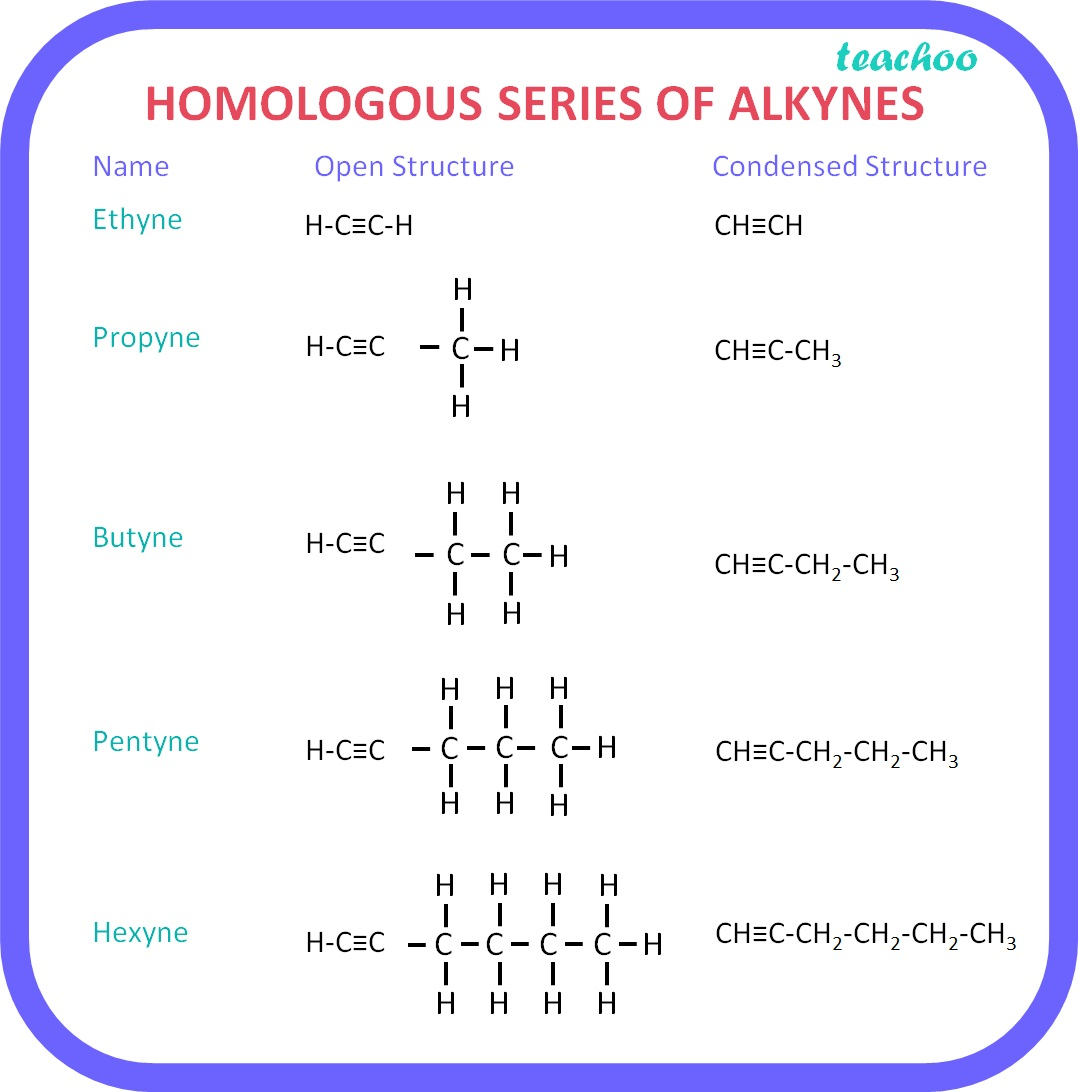Concepts

Class 10
Chapter 4 Class 10 - Carbon and its Compounds

## What is a Homologous Series?

• It is a series of organic compounds having similar structures and similar chemical properties .
• 2 successive compounds differ by a CH 2 in a series.
• For Example, Alkanes, Alkenes and Alkynes are homologous series.

### Characteristics of Homologous Series:

• Difference between 2 successive compounds of any homologous series is 14u and a CH 2  group .
• It is because the mass of carbon is 12u and hydrogen is 1u . Hence mass of CH 2 will be 12+1*2=12+2=14u.

Example:

• Mass of Methane (CH 4 ): 12 + 1 × 4 = 12 + 4 = 16u
• Mass of Ethane (C 2 H 6 ): 12 × 2 + 1 × 6 = 24 +6 = 30u
• Difference in Mass = 30 - 16 = 14u

### Homologous Series of Alkanes:

• If we compare  Methane (CH 4 ) and Ethane (C 2 H 6 ):
• Ethane has 1 Carbon atom more than Methane. Also, Ethane has 2 Hydrogen atoms more than Methane. Hence, we can say that Ethane and Methane are successive compounds which differ by CH 2 group .
• All members of a series can be represented by a general formula : C n H 2n+2 .

### Homologous Series of Alkenes:

• If we compare Ethene (C 2 H 4 ) and Propene (C 3 H 6 ) :

Propene has 1 Carbon atom and 2 Hydrogen atoms more than Ethene.

Hence, we can say that Propene and Ethene  are successive compounds which differ by CH 2 group.

• All the alkenes can be represented by the general formula : C n H 2n .

###googletag.cmd.push(function() { googletag.display('div-gpt-ad-1669298377854-0'); });

• If we compare Ethyne (C 2 H 2 ) and Propyne (C 3 H 4 ):
• The latter ha s 1 carbon atom and 2 hydrogen atoms more as compared to the former
i.e., propyne. Therefore, we can say that
Propene and Ethene are two successive compounds which differ by CH 2 group.
• All the alkynes can be represented by the general formula: C n H 2n-2 .
• All compounds of a chemical series show similar chemical properties . Example: Methane and ethane are both flammable gasses.
• As the molecular mass increases in any homologous series, a gradation in physical properties is seen. This is because the melting and boiling points increase with increasing molecular mass .
• Other physical properties such as solubility in a particular solvent also show a similar gradation.
• But the chemical properties, which are determined solely by the functional group, remain similar in a homologous series .

Get live Maths 1-on-1 Classs - Class 6 to 12